• VB程序设计for循环语句说课稿PPT课件.pptx
• for循环 1.语法结构 2.执行顺序 3.注意说明 （一）语法结构： For 循环变量=初值 to 终值【step 步长】 【循环体】 【Exit for】 Next 【循环变量】【，循环变量】 计算1累加到100的和 Dim i as integer '声明变量i...
for循环
1.语法结构
2.执行顺序
3.注意说明
（一）语法结构： For 循环变量=初值 to 终值【step 步长】 【循环体】 【Exit for】 Next 【循环变量】【，循环变量】
计算1累加到100的和

Dim i as integer         '声明变量i
Dim sum as long          '声明变量sum
i=1:sum=0                '将1赋值给变量i，将0赋值给变量sum
For  i=1 to 100 step 1   '循环1-100之间的数，循环完一次就将i+1
sum=i+sum        '将sum+i的值赋值给新的sum
Next i
print sum                '循环结束后输出结果sum


执行顺序
①将1赋值给i，然后判断i是否在1-100区间内 ②如果在，执行循环体内的sum=sum+i ③由于step 1，所以相当于变量i=i+1 ④再继续判断是否在1-100区间内 ⑤一直判断到 i>100 跳出循环，输出sum
说明 1、步长：默认为1时可以省略，不可为0 2、循环次数：Int（终值-初值)/步长+1 3、For-Next循环次数可以使用嵌套循环
for循环的嵌套使用
Dim i as integer
Dim j as integer
i=1:j=1
For  i=1 to 2 step 1
print "*"
For j=1 to 2 step 1
print "#"
Next j
Next i
print sum

for嵌套的例子 九九乘法表
Dim i as Integer
Dim j as Integer
i=1:j=1
For i=1 to 9
For j=1 to i  '注意一行里面输出的列的个数不能超过行的个数；所以终值是i所以第一个循环跟第二个的循环次数相同
Print i & "*" & j & "=" & i * j;space(2);   ’在输出语句后面加分号（;）代表着 连着输出不换行
Next j
print   '把第一行循环的结果全部输出在一行上 ，再换行
Next i

展开全文• VB程序设计练习题（一） 文章目录 目录 前言 内容 1、十进制转二进制数 2、程序实现功能：小明过年要给他的40位同学各寄一张贺卡，贺卡的价格有3元、2元和1元三种，他一共有100元，怎样购买正好把钱用完。把...
VB程序设计练习题（一）
文章目录
目录
VB程序设计练习题（一）
文章目录
前言
内容
1、十进制转二进制数
2、购买贺卡问题
三、用滚动条改变标签颜色
4.猴子吃桃，递推举法
5、比赛最高分与最低分
6、人口问题
7.二维数组矩阵输出
8.二维数组4*4矩阵输出
9、（二维数组）求二维数组四周元素的和
10.输出5*5矩阵求对角线之和
11、输出三角形
12.单击窗体，利用双重循环输出如下的图案。
13、输出连续和为1250的正整数范围
14、求Π的值
15、单词首字母大写
16、辗转相除法求最大公约数
17、累乘与累加
18、双胞胎数
19、for语句输出三角形
20、杨辉三角形

前言
备考技能考的一些陌生的VB练习题
难度比较大
有什么问题可以在评论区发表

内容
1、十进制转二进制数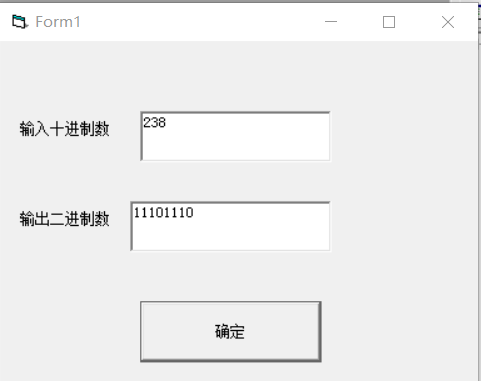答案:
Private Sub Command1_Click()
Dim i As Integer
a = Val(Text1.Text)
If a >= 0 And a <= 255 Then
For i = 1 To 8
If a Mod 2 = 1 Then
a = a \ 2
b = b & "1"
Else
a = a / 2
b = b & "0"
End If
Next
Else
MsgBox "请输入正确的十进制数："
End If
Text2.Text = StrReverse(b)
End Sub

2、购买贺卡问题
程序实现功能：小明过年要给他的40位同学各寄一张贺卡，贺卡的价格有3元、2元和1元三种，他一共有100元，怎样购买正好把钱用完。把所有可能的组合显示出来。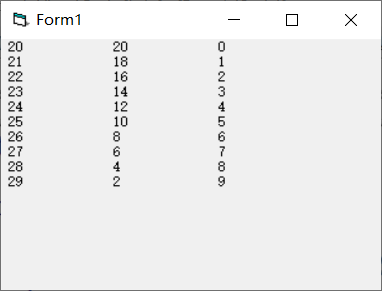答案：
Private Sub Form_Click()
dim a,b,c,d,e,f
a = 3
b = 2
c = 1
For d = 0 To 34
For e = 0 To 50
For f = 0 To 100
If a * d + b * e + c * f = 100 And d + e + f = 40 Then
Print d; Space(5); e; Space(5); f
End If
Next
Next
End Sub

三、用滚动条改变标签颜色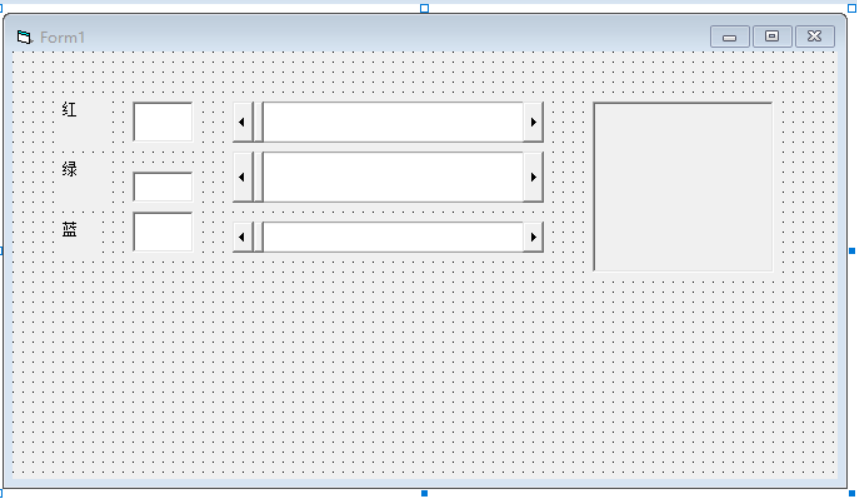答案:

Private Sub HSB_Change()
Text3.Text = HSB.Value
Label4.BackColor = RGB(Val(Text1.Text), Val(Text2.Text), Val(Text3.Text))
End Sub

Private Sub HSB_Scroll()
Text3.Text = HSB.Value
Label4.BackColor = RGB(Val(Text1.Text), Val(Text2.Text), Val(Text3.Text))
End Sub

Private Sub HSG_Change()
Text2.Text = HSG.Value
Label4.BackColor = RGB(Val(Text1.Text), Val(Text2.Text), Val(Text3.Text))
End Sub

Private Sub HSG_Scroll()
Text2.Text = HSG.Value
Label4.BackColor = RGB(Val(Text1.Text), Val(Text2.Text), Val(Text3.Text))
End Sub

Private Sub HSR_Change()
Text1.Text = HSR.Value
Label4.BackColor = RGB(Val(Text1.Text), Val(Text2.Text), Val(Text3.Text))

End Sub

Private Sub HSR_Scroll()
Text1.Text = HSR.Value
Label4.BackColor = RGB(Val(Text1.Text), Val(Text2.Text), Val(Text3.Text))
End Sub

4.猴子吃桃，递推举法
（要以上一个本身做铺垫才能求出下一个的值的，如果把i设为循环值，在循环中的i只是它的下标签，在循环中不需要把i当系数，也就是说循环中不出现i）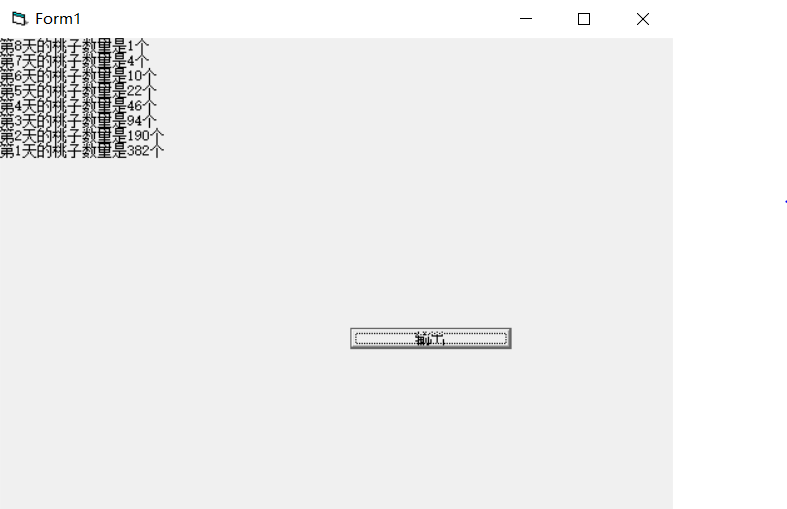答案:
Private Sub Command1_Click()
x = 1
Print "第8天的桃子数量是1个"
For i = 7 To 1 Step -1
x = (x + 1) * 2         #循环中不需要出现i
Print "第" & i & "天的桃子数量是" & x & "个"
Next
End Sub

5、比赛最高分与最低分
某次歌曲大奖赛，有7个评委对某个参赛者的打分数，按照去掉一个最高分和最低分的计算办法，求出该参赛者的平均得分以及最高分和最低分。
答案：
Private Sub Command1_Click()
Dim a(1 To 7), i, s, b As Integer
s = 0
For i = 1 To 7      ‘先定义i的for循环，用对话框的方式输入每个评委的得分。
a(i) = Val(InputBox("第" & i & "个评委得分"))
s = s + a(i)         ‘把所有评委的得分加起来
Next
Min = a(1)          ‘先定义Min等于a(1)的值
For i = 1 To 7
If a(i) > Max Then     ‘让每个数都于Max比较，若大于则替换值。（Max初始最为0）
Max = a(i)
End If
Next
For i = 1 To 7          ‘让每个数都于Min比较，若小于则替换值。（Min=a（1））
If a(i) < Min Then
Min = a(i)
End If
Next
s = s - (Max + Min)        ‘最后减掉最大值与最小值
b = s / 5                  ‘求平均值
Print "最大值" & Max & vbCrLf & "最小值" & Min & vbCrLf & "去掉最高分与最低分的平均值是" & b     ‘输出
End Sub

6、人口问题
我国目前有13亿的人口，按人口年增长率0.8%计算，多少年后我国人口将超过26亿
答案：
Private Sub Command1_Click()
a = 13      ‘先定义a为13
s = 0
Do While a <= 26
a = a * (1 + 0.008)
s = s + 1
Loop
Print s
End Sub

7.二维数组矩阵输出
（二维数组）随机生成一个整型的二维数组，范围在[10,20]之间，以下三角形形式输出该数组。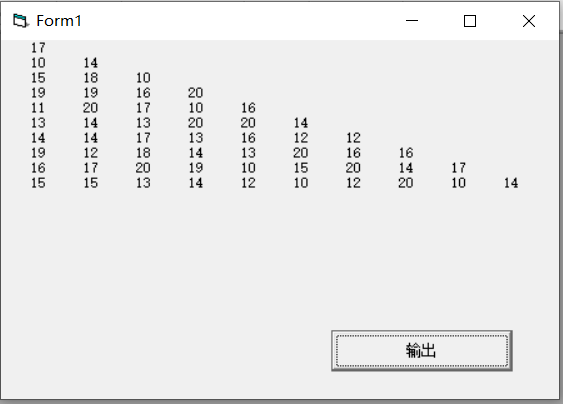答案：
Private Sub Command1_Click()
Dim a(1 To 10, 1 To 10) As Integer
For i = 1 To 10
For j = 1 To 10
a(i, j) = Int(Rnd * (20 - 10 + 1) + 10)
Next
Next
For i = 1 To 10
For j = 1 To i
Print Spc(3); a(i, j);
Next
Print
Next
End Sub

8.二维数组4*4矩阵输出
（二维数组）利用随机函数生成一个4*4的矩阵（即二维数组），范围是[20,50]内的整数，输出该矩阵所有数据的之和。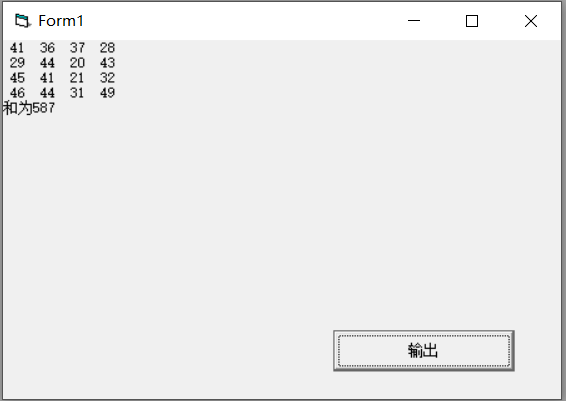答案：
Private Sub Command1_Click()
Dim a(1 To 4, 1 To 4) As Integer
For i = 1 To 4
For j = 1 To 4
a(i, j) = Int(Rnd * (50 - 20 + 1) + 20)
Print a(i, j);
s = s + a(i, j)
Next
Print
Next
Print "和为" & s
End Sub

9、（二维数组）求二维数组四周元素的和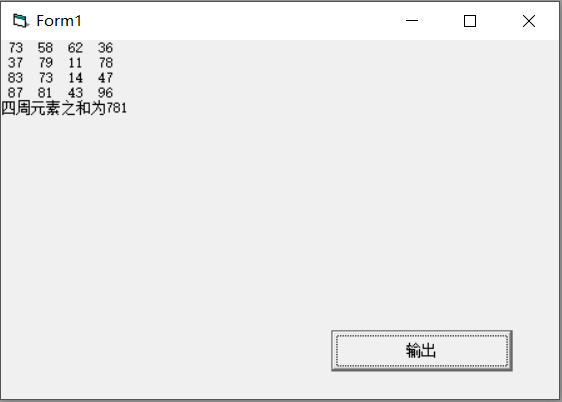答案：
Dim a(4, 4) Private Sub Command1_Click() For i = 1 To 4
For j = 1 To 4
If i = 1 Or j = 1 Or i = 4 Or j = 4 Then
Sum = Sum + a(i, j)
End If
Next
Next
Print "四周元素之和为" & Sum
End Sub
Private Sub Form_Click() For i = 1 To 4     For j = 1 To 4         a(i, j) = Int(Rnd * (99 - 10 + 1) + 10)         Print a(i, j);              Next     Print      Next
End Sub

10.输出5*5矩阵求对角线之和
(二维数组)用二维数组加双重循环输出5*5的方阵，每个数组的值的区间[10,99]之间，并且输出对角线的值的之和。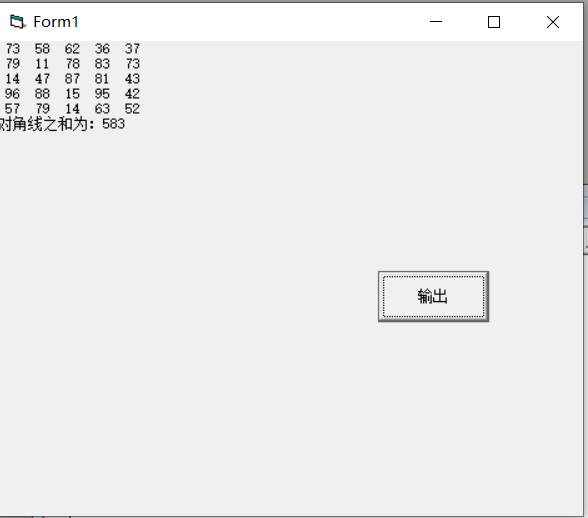答案：
Private Sub Command1_Click()
Dim a(1 To 5, 1 To 5) As Integer
For i = 1 To 5
For j = 1 To 5
a(i, j) = Int(Rnd * (99 - 10 + 1) + 10)
If i = j Then
s = s + a(i, j)
End If
If i + j = 6 Then
b = b + a(i, j)
End If
Print a(i, j);
Next
Print
Next
Print "对角线之和为：" s + b - a(3, 3)
End Sub

11、输出三角形
利用双重循环输出以下的图案。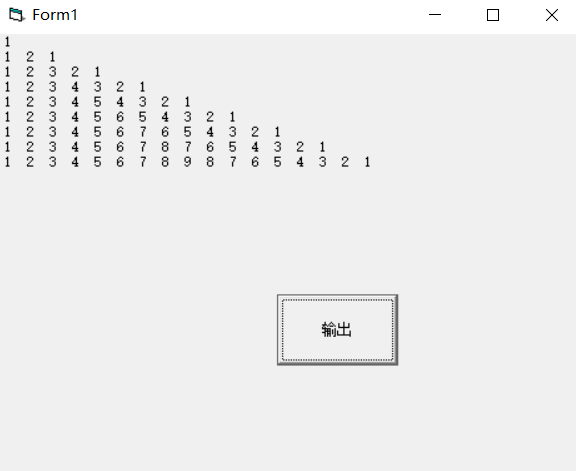答案：
Private Sub Command1_Click()
For i = 1 To 9
For j = 1 To I         以上是先输出一个直角三角形的1到i的数。（循环会先把上面的j
Print j;              循环全部输出出来再输出下一个循环，即e
Next
If i >= 2 Then          这里开始输出e的循环 i-1到1的数。
For e = i - 1 To 1 Step -1
Print e;
Next
End If
Print
Next
End Sub

12.单击窗体，利用双重循环输出如下的图案。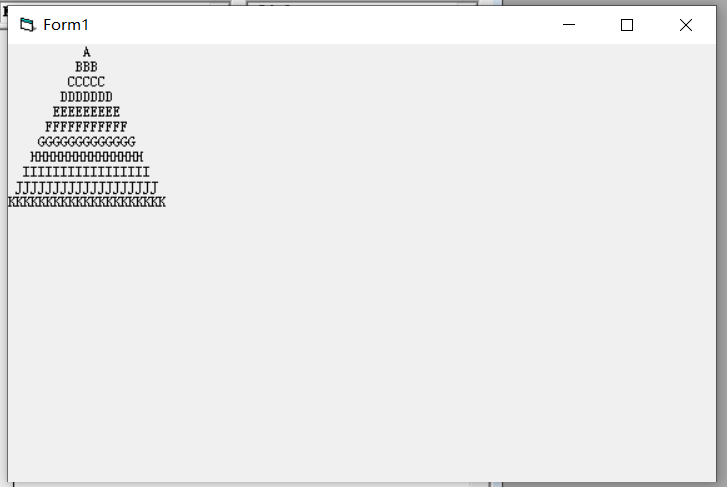答案：
Private Sub Form_Click()
For i = 65 To 75
Print Space(75 - i);
For j = 129 To 2 * i - 1
Print Chr(i);
Next
Print
Next
End Sub

13、输出连续和为1250的正整数范围
在窗体上创建一个命令按钮，标题为“计算并输出”；程序运行后，如果单击命令按钮，程序将计算500以内两个数之间（包括开头和结尾的数）所有连续数的和为1250的正整数，并在窗体上显示出来，这样的数有多组，程序输出每组开头与结尾的正整数，并用“~”连接起来，如图所示。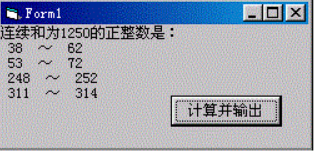答案：
Private Sub Command1_Click()
Print "连续和为1250的正整数是："
For i = 1 To 500   首先定义一个双重循环。I是1到500，j是i到500，所以j的循环要取
For j = i To 500    决于i。
s = s + j           累加
If s > 1250 Then   看看累加的s大于1250吗，若大于则停止j循环，接着i循环。
Exit For
ElseIf s = 1250 Then 若等于s后，则输出i循环所用的值，也就是开头，再输出j循环的值，
Print i; "~"; j        也就是结尾。
Exit For           这里还是要停止循环，
End If
Next
s = 0            s=0，初始化，让下一次循环用。
Next
End Sub

14、求Π的值
布局如下图。单击按钮，根据下面的公式计算Π的值：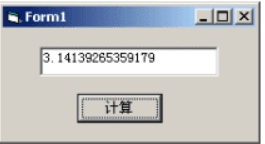Π/4=1-1/3+1/5-1/7+…….1/10001
用do….while 实现程序。
答案：
Private Sub Command1_Click()
Dim r , s, i
r = 0
s = 1
i = 1
Do While 1 / i >= 0.0001
r = r + s / i
i = i + 2
s = -s
Loop
Text1.Text = r * 4
End Sub

15、单词首字母大写
按照如下图布置，程序运行后，单击按钮，文本框中的每个单词的首字母变成大写。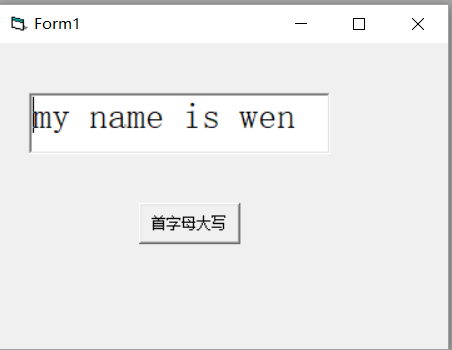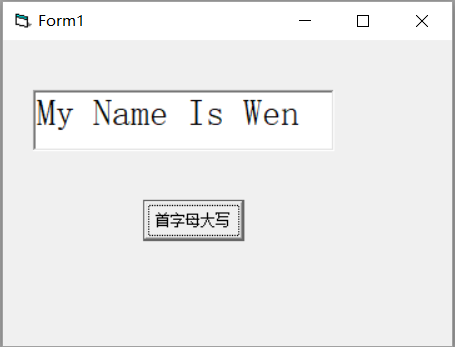答案：
Private Sub Command1_Click()
Dim i, a, f, c
f = 0                                首先定义f=0，c=””
c = ""
For i = 1 To Len(Text1.Text)              循环i=1到text1.text的长度
a = Mid(Text1.Text, i, 1)                 a=循环等于每个字母
If i = 1 Then                          当第一个字母的时，第一个字母转化为大写
a = UCase(a)
End If
If f = 1 Then                         当f=1时，这里只有运行下面那个a=” ”，才能触发
a = UCase(a)                         当运行下面的a=” “的时候，f才会为1。也就是说
f = 0                                空格的后一项字母为大写。
End If
If a = " " Then
f = 1
End If
c = c & a
Next
Text1.Text = c
End Sub

16、辗转相除法求最大公约数
创建3个文本框，计算text1和text2的正整数的最大公约数，并显示在text3中。
（要求使用do…….loop语句来实现）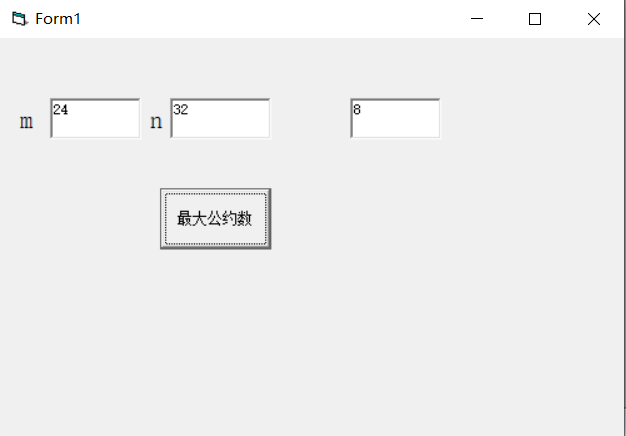答案：
Private Sub Command1_Click()
m = Val(Text1.Text)
n = Val(Text2.Text)
If n > m Then              t = n              n = m              m = t         End If
Do
r = m Mod n
If r = 0 Then Exit Do
m = n
n = r
Loop
Text3.Text = n
End Sub

17、累乘与累加
点击窗体，已知s=1+1/3!+1/5!+1/7!+1/9!.将s的结果输出到窗体上。使用do while….loop语句完成程序。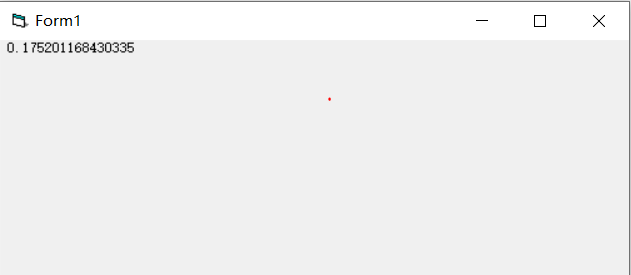答案：
Private Sub Form_Click()
Dim i As Integer
i = 1           这相当于for的初始值
s = 1
Do While i <= 9  这相当于for的结束值
i = i + 1         公差，相当于for循环里的step（必须定义）
s = s * i
If i Mod 2 = 1 Then
p = p + 1 / s
End If
Loop          这里相对于next
Print p
End Sub

18、双胞胎数
若两个素数之差为2，则称这两个素数为双胞胎数。求出[200，1000]之内有多少对双胞胎数。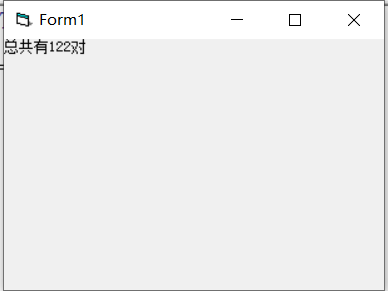答案：
Private Sub Form_Click()
For i = 200 To 1000
f = 0
For j = 2 To i - 1
If i Mod j = 0 Then
f = 1
Exit For
Else
f = 0
End If
Next
If f = 0 Then            1.当求出200到1000之间第一个素数时（从小到大），就循环下
For e = 200 To 1000        组素数。
n = 0
For a = 2 To e - 1
If e Mod a = 0 Then
n = 1
Exit For
Else
n = 0
End If
Next
If f = 0 Then        2.当是素数时。
If i + 2 = e Then .则判断第一组中的素数是否加2等于第二组中的素数。（相差为2
s = s + 1  ，若满足则s加1.
Exit For  ，退出循环（因为已经找到了，就不可能有第二个一样的）
End If
End If
Next
End If
Next
Print "总共有" & s & "对"
End Sub

19、for语句输出三角形
（新思路）用for语句输出如下图案。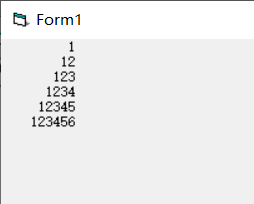答案：
Private Sub Command1_Click()
Dim i, j As Integer
a = "123456"            先定义一个a字符串是1到6
For i = 1 To 6
Print Space(10 - i); Mid(a, 1, i)  利用mid函数来选择
Next
End Sub

20、杨辉三角形
编写适当的事件过程，在窗体上输出相对应行数的杨辉三角形，点击窗体弹出inputbox对话框，在对话框中输入行数，打印出如下图所示的杨辉三角形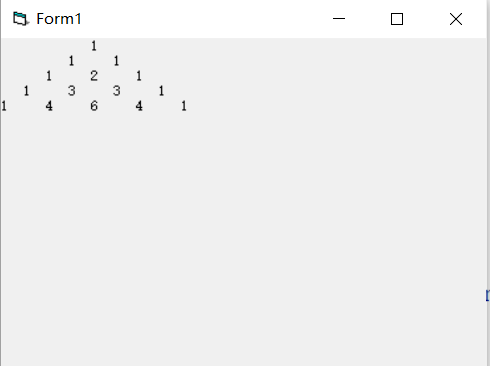答案：
Private Sub Form_Click()
Dim i As Integer, j As Integer
Dim a As Integer
a = Val(InputBox("请输入行数"))
Dim c(9, 9) As Integer
If a > 0 Then
For i = 1 To a
Print Space(3 * a - 3 * i);
For j = 1 To i
If i = 1 Or j = i Then       ‘当为三角形两边的时候，输出1
c(i, j) = 1
End If
If i = 2 Or j = i - 1 Then              ‘当为三角形的两边里面一个的时候输出行数-1
c(i, j) = i - 1
End If
If i > 2 And j < i - 1 Then                    ‘否则都以自身上面一行对角线两个数的和。
c(i, j) = c(i - 1, j - 1) + c(i - 1, j)
End If
Print c(i, j) & "     ";                       ‘输出
Next
Print
Next
End If
End Sub

点赞、关注
展开全文编程语言
• ## VB程序设计练习题

千次阅读 2021-05-15 14:03:35
这是我备考技能考的一些VB练习题。（难度稍微有点大哦） 想要更多VB练习题点击我的头像，看我发表我文章就可以看到了。 答案是小编自己写的，也许会有错。 内容 一、明文密文转换。 在上面的文本框中输入的英文...
文章目录
目录
文章目录
前言
内容
一、明文密文转换。
二、大小写互相转换。
三、三角形的综合问题。
五、标签左右移动。
六、相邻数加起来为素数。
七、通项分母(累加).
八、判断降序数。
九、产生数组，求最大数。
十、奇偶排序。
总结：

前言
这是我备考技能考的一些VB练习题。（难度稍微有点大哦）
想要更多VB练习题点击我的头像，看我发表我文章就可以看到了。
答案是小编自己写的，也许会有错。

内容
一、明文密文转换。
在上面的文本框中输入的英文字母（称为”明文")加密，加密结果（称为"密文")显示在下面的文本框中。加密的方法是：选中一个单选按钮，单击“加密”按钮后，根据选中的单选按钮后面的数字n，把明文中的每个字母改为它们后面第n个字母（"z"后面的字母认为是"a"，"Z"后面的字母认为是"A"），如图所示。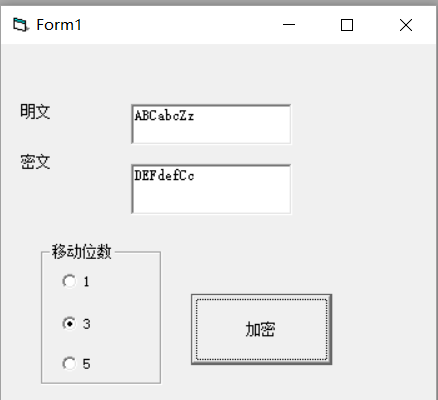答案：
Private Sub Command1_Click() For i = 1 To Len(Text1.Text)       If Option1.Value = True Then             a = Mid(Text1.Text, i, 1)             b = Asc(a) + 1       End If       If Option2.Value = True Then             a = Mid(Text1.Text, i, 1)             b = Asc(a) + 3       End If       If Option3.Value = True Then             a = Mid(Text1.Text, i, 1)             b = Asc(a) + 5       End If       If b > 122 Then             b = b - 26       End If       If b > 90 And b < 97 Then             b = b - 26       End If       Text2.Text = Text2.Text & Chr(b)
Next
End Sub

二、大小写互相转换。
如图所示创建界面（一个文本框，一个按钮），程序运行后，单击“转换”按钮，可以text1中的大写字母转换为小写，把小写字母转换为大写。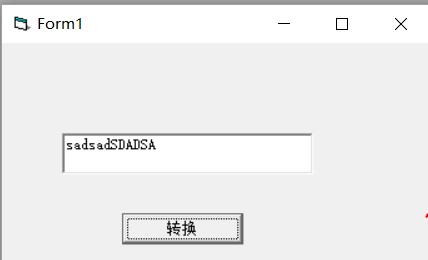答案：
Private Sub C1_Click()
For i = 1 To Len(Text1.Text)
a = Mid(Text1.Text, i, 1)              ‘一个一个选择text1中的字符
For j = 97 To 122
If a = Chr(j) Then                   ‘判断是不是小写字母
f = 0                             ‘是的话f=0
Exit For                          ‘并且要停止循环，不然会一直循环下去导致f=1。
Else                             ‘否则f=1，也就是大写字母
f = 1
End If
Next
If f = 0 Then                        ‘如果是f=0（也就是字母为小写的情况）
a = UCase(a)                        ‘就转大写
End If                             ‘结束
If f = 1 Then                         ‘如果f=1（也就是大写的情况）
a = LCase(a)                         ‘就转小写
End If
b = b & a                           ‘全部a都赋值给b
Next
Text1.Text = b                         ‘text1输出b
End Sub

三、三角形的综合问题。
根据给定的图形的三边的边长来判断图形的类型。若为三角形则同时计算出何种三角形，及三角形的周长和面积。要求完成”判断并计算”按钮的如下功能：
1、判断输入的条件是否为三角形，若是三角形则在Text1中显示“是三角形”；在Text2中显示是何种三角形。
2、单击“清除重来”按钮可以将所有的显示框清空，且按钮本身变为不可选取状态。当单击“判断并计算”之后重新恢复为可选状态。
提示：1.三角形存在的条件为任一边不为0且任两边之和大于第三边。
2.若一边具有a^2+b^2=c^2，则为直角三角形；若所有边具有a^2+b^2>c^2,则为锐角三角形；若一边具有a^2+b^2<c^2,则为钝角三角形。
3、三角形的面积公式.已知三角形三边a,b,c，则
（海伦公式）
（p=(a+b+c)/2）
S=sqrt[p(p-a)(p-b)(p-c)]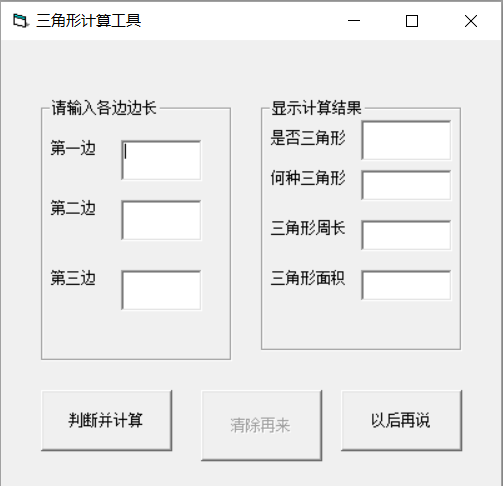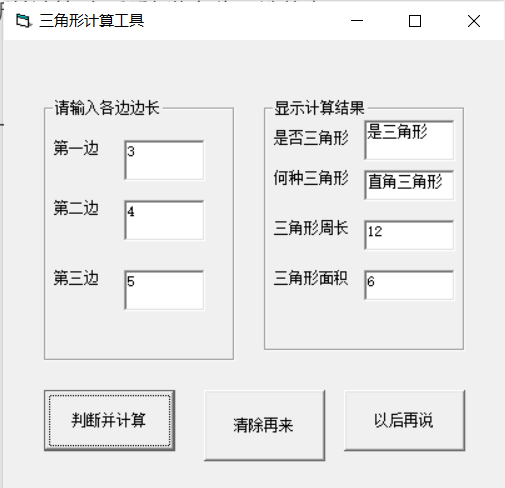答案：
Private Sub Command1_Click()
a = Val(Text1.Text)
b = Val(Text2.Text)
c = Val(Text3.Text)
Command2.Enabled = True
If a + b > c And a + c > b And c + b > a Then
Text4.Text = "是三角形"
Else
Text4.Text = "不是三角形"
End If
If Text4.Text = "是三角形" Then
If a ^ 2 + b ^ 2 = c ^ 2 Or a ^ 2 + c ^ 2 = b ^ 2 Or b ^ 2 + c ^ 2 = a ^ 2 Then
Text5.Text = "直角三角形"
End If
If a ^ 2 + b ^ 2 > c ^ 2 And a ^ 2 + c ^ 2 > b ^ 2 And b ^ 2 + c ^ 2 > a ^ 2 Then
Text5.Text = "锐角三角形"
End If
If a ^ 2 + b ^ 2 < c ^ 2 Or a ^ 2 + c ^ 2 < b ^ 2 Or b ^ 2 + c ^ 2 < a ^ 2 Then
Text5.Text = "钝角三角形"
End If
Text6.Text = a + b + c
Text7.Text = (1 / 4) * Sqr((a + b + c) * (a + b - c) * (a + c - b) * (b + c - a))
End If
End Sub
Private Sub Command2_Click()
Text1.Text = ""
Text2.Text = ""
Text3.Text = ""
Text4.Text = ""
Text5.Text = ""
Text6.Text = ""
Text7.Text = ""
End Sub
Private Sub Command3_Click()
End
End Sub

四、杨辉三角形。
编写适当的事件过程，在窗体上输出相对应行数的杨辉三角形，点击窗体弹出inputbox对话框，在对话框中输入行数，打印出如下图所示的杨辉三角形
会做这题，说明二维数组输出掌握很好。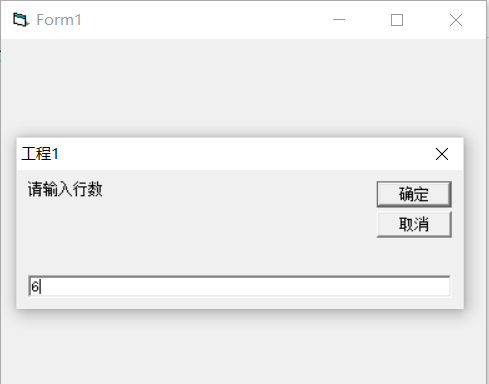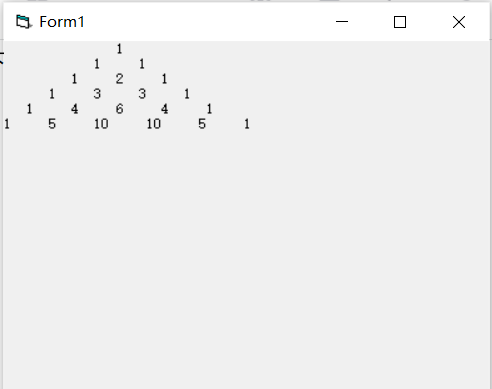答案：
Private Sub Form_Click()
Dim i As Integer, j As Integer
Dim a As Integer
a = Val(InputBox("请输入行数"))
Dim c(9, 9) As Integer
If a > 0 Then
For i = 1 To a
Print Space(3 * a - 3 * i);
For j = 1 To i
If i = 1 Or j = i Then       ‘当为三角形两边的时候，输出1
c(i, j) = 1
End If
If i = 2 Or j = i - 1 Then              ‘当为三角形的两边里面一个的时候输出行数-1
c(i, j) = i - 1
End If
If i > 2 And j < i - 1 Then                    ‘否则都以自身上面一行对角线两个数的和。
c(i, j) = c(i - 1, j - 1) + c(i - 1, j)
End If
Print c(i, j) & "     ";                       ‘输出
Next
Print
Next
End If
End Sub

五、标签左右移动。
点击开始按钮，标签右移动，到达右边则向左移动，到达左边则向右移动，点击停止按钮则停止移动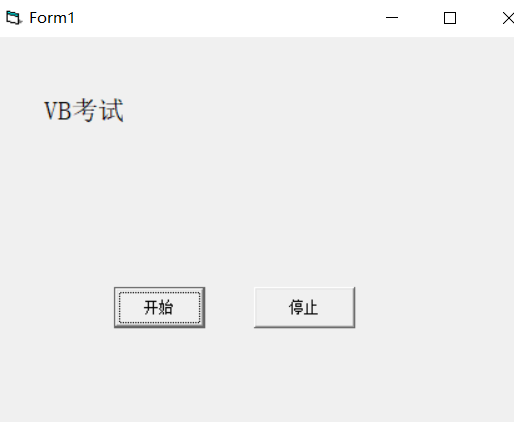答案：
Dim a
Private Sub Command1_Click()
Timer1.Enabled = True
End Sub
Private Sub Command2_Click()
Timer1.Enabled = False
End Sub
Timer1.Enabled = False
a = 0
End Sub
Private Sub Timer1_Timer()
If Label1.Left >= Val(Form1.Width) - Val(Label1.Width) Then
a = 1
End If
If Label1.Left <= 0 Then
a = 0
End If
If a = 1 Then
Label1.Left = Label1.Left - 100
End If
If a = 0 Then
Label1.Left = Label1.Left + 100
End If
End Sub

六、相邻数加起来为素数。
本程序用于求1~10中相邻数之和是素数的所有自然数对。
例如：1和2为相邻数并且1+2=3，3为素数，则输出1和2.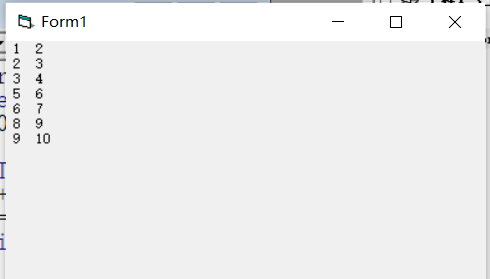答案：
Private Sub Form_Click()
Dim i As Integer
For i = 1 To 10
f = 0
For j = 2 To i - 1
If (i + (i + 1)) Mod j = 0 Then
f = 1
Exit For
Else
f = 0
End If
Next
If f = 0 Then
Print i; i + 1
End If
Next
End Sub

七、通项分母(累加).
下面程序的功能是计算，若通项分母的值>=200，停止计算（正确答案是S=1.409524）
S=1+1/1*3 + 1/1*3*5 +1/1*3*5*7…..
答案：
Private Sub Form_Click()
Dim i As Integer
Dim n As Integer
i = 1
n = 1
Do
If n > 200 Then
Exit Do
Else
Print i
Sum = Sum + (1 / n)
End If
i = i + 2
n = n * i
Loop
Print Sum
End Sub
第二种：
Private Sub Form_Click()
Dim i As Integer
Dim n As Integer
i = 1
n = 1
Do While n <= 200
Sum = Sum + (1 / n)
i = i + 2
n = n * i
Loop
Print Sum
End Sub

八、判断降序数。
在文本框中输入一个整数数，判断是否为降序数。（降序数是高位的数比低位的数要大，依次降序，如321，654，9876等。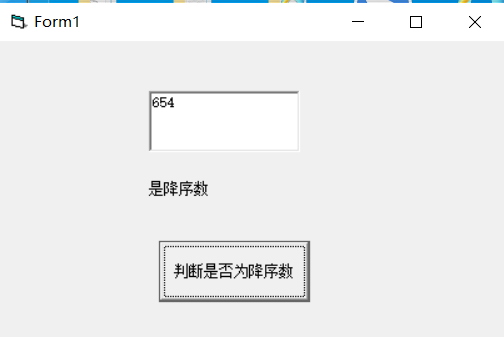答案：
Private Sub Command1_Click()
Dim a As String
a = Text1.Text
f = 0
If Val(a) > 0 And Val(a) = Int(Val(a)) Then
For i = 2 To Len(a)
If Val(Mid(a, i, 1)) <= Val(Mid(a, i - 1, 1)) Then   '当题目没给给定多少位，就是说不能使用数 组来做，则可以直接吧mid当做数组
f = 1
Else
f = 0
Exit For
End If
Next
If f = 1 Then
Label1.Caption = "是降序数"
Else
Label1.Caption = "不是降序数"
End If
Else
MsgBox "请输入正整数"
End If
End Sub

九、产生数组，求最大数。
点击窗体，用inputbox输出9个整数，放入数组a中并输出在窗体上，本程序按序号依次将（a1,a2,a3）,(a2,a3,a4),…..(a9,a1,a2),九组元素分别相加（每组3个元素），生产新数组b并输出在窗体上，然后找出b数组中的最大元素并输出在窗体上。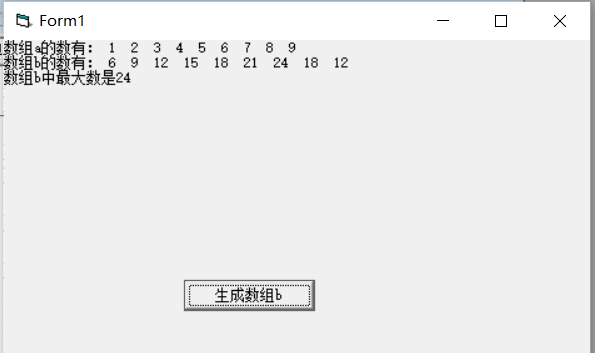答案：
Dim a(1 To 9) As Integer
Dim b(1 To 9) As Integer
Private Sub Command1_Click()
Dim c1, c2, c3
Print "数组b的数有：";
For i = 1 To 9
c1 = i
c2 = i + 1
c3 = i + 2
If c2 > 9 Then
c2 = c2 - 9
End If
If c3 > 9 Then
c3 = c3 - 9
End If
b(i) = a(c1) + a(c2) + a(c3)
Print b(i);
Next
Print
For i = 1 To 9
If b(i) > Max Then
Max = b(i)
End If
Next
Print "数组b中最大数是" & Max
End Sub
Private Sub Form_Click()
Print "数组a的数有：";
For i = 1 To 9
a(i) = Val(InputBox("请输入第" & i & "个数"))
Print a(i);
Next
Print
End Sub

十、奇偶排序。
随机生成9个数（范围为1~99）的数列，输出在窗体上，新数列的规则是：序列左边是奇数，序列右是偶数，奇、偶数分别从序列的两端开始依次向序列中间排放。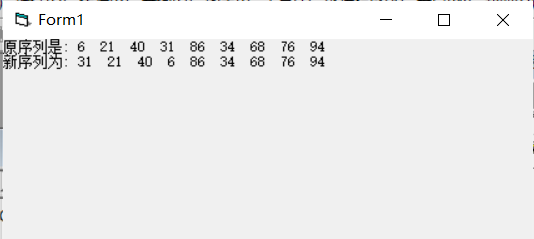答案：
Private Sub Form_Click()
Dim a(1 To 9)
Dim b(1 To 9)
Randomize
Print "原序列是:";
For i = 1 To 9
a(i) = Int(Rnd * (100 - 1 + 1) + 1)
Print a(i);
Next
Print
Print "新序列为:";
For i = 1 To 9
For j = 9 To i + 1 Step -1
If a(i) Mod 2 = 0 And a(j) Mod 2 <> 0 Then
t = a(i)
a(i) = a(j)
a(j) = t
End If
Next
Print a(i);
Next
End Sub

总结：
这是我发的第三份VB练习题，有同学能在不看答案的情况下能把三份的题全部做出来，说明你的VB这一块非常强了，在班级里应该是大神级的人物，考试对于你来说就是送分题。
当然有些基础不是很好，有些题是做不出来的同学也不要灰心，但是一定要去多自己尝试着做，别看一下题目就去看答案，这是没有用的。实在是想不出来的情况下在看看答案，理清答案的做题思路。千万不要去背答案哦，要理清答案思路，以后碰见了类似的题能自己做出来。
我在做这些题的时候是没有答案的，这些题的答案是我自己想出来的，我遇到了难题都是考自己想出来的，有些难题我花了一两个小时才写出来。
一开始我也是做题比较慢，经过不断的练习，做题速度就慢慢快了起来。
因为我发的题都是一些比较难的综合题，不会做也很正常，当然要是会做，那就更好了。
遇到一些不懂的地方可以私信或者评论哦！

展开全文编程语言 后端 经验分享
• 实验四 循环结构程序设计 (解答 ) 1改错题 1下列程序的功能为求 1 100 之和和值为 5050 并输出请纠正程序中存在 错误使程序实现其功能程序以文件名 sy4_1.c 存盘 #include <stdio.h> main) { int i,sum=0;...
• 运行效果： 程序代码： Private Sub Command1_Click()  Dim a()  n = 10    'Val(Text1.Text)  Me.Cls  ReDim a(n + 1, n + 1)  For i = 1 To n    For j = 0 To i    a(1...

运行效果：程序代码：

Private Sub Command1_Click()

Dim a()

n = 10           'Val(Text1.Text)

Me.Cls

ReDim a(n + 1, n + 1)

For i = 1 To n

For j = 0 To i

a(1, i) = 1

a(i, i) = 1

a(i + 1, j + 1) = a(i, j) + a(i, j + 1)

Print a(i, j);

Next j

Print

Next i

End Sub

学习总结：

算法的五大特性：

1、有穷性

2、确定性

3、可行性

4、输入

5、输出


展开全文• while循环九九乘法表、do.while循环九九乘法表、for循环_九九乘法表
• 文章目录 前言 本文是一些基础题给同学们练习练习，认为太简单的同学可以点击我头像看其他VB题。 内容编程语言
• 《高级语言程序设计》第四章 循环结构程序设计（说课PPT），我们老师（副教授）的PPT，供有识别之士参考。
• Chapter1 算法与程序设计 1.1计算机数据的编码 数值数据形式：2/8/10/16进制 编码：“0”“1”二进制表示数据，一般采用BCD码表示十进制数据 数值的表示：真值&机器数 真值：保留符号（+/-） 机器数：+/-...
• 利用vb打印菱形的星号，多重循环实现，详细的源代码打包中
• VB允许你将不同类型的循环For和Do循环）“嵌套”在同一个过程里。当你编写循环嵌套时，请确保每个内部的循环在外部循环里面已经完成。另外，每个循环都必须有其自己独特的计数器变量。如果使用循环嵌套，你可以更...excel vba
• 编写一个能将任意两个文件的内容合并的程序程序界面由读者由自由设计。 解题，在窗体建立一个按钮控件，假定C盘已经有两个要合并的文件text1.dat和text2.dat,代码如下： Private Sub Command1_Click() Dim char ...实验
• ## VB程序设计及应用

热门讨论 2012-11-26 14:07:12
中文名: VB程序设计及应用 作者: 李淑华图书分类: 软件 资源格式: PDF 版本: 文字版 出版社: 高等教育出版社书号: 7-04-014767-X发行时间: 2004年04月 地区: 大陆 语言: 简体中文 简介: 内容简介： 本书是新...
• Visual Basic 6.0 程序设计教程 第三章结构化程序设计与数组 重点 选择结构 IF结构情况选择结构 循环结构 For/NextWhile/Wend Do/Loop循环 多重循环 双重循环 数组 一维和二维数组 2 1 1 1 1 1 1 40 求算式 前 项的...
• 赋值语句是程序设计中的基本语句，它可以把指定的值赋给某个变量或者某个对象的属性。 （一）给变量赋值 Dim a As Integer a=2 （二）给对象的属性赋值 1. 界面设计 2. 代码 Private Sub Command1_Click()...
• 这里使用的是《vb程序设计教程（第四版）——龚沛曾》： 实验3（主要考察分支选择结构。1—7考察select和if语句，8用到choose函数，9—11以控件option和check为主） 3.1： Option Expl...
• For循环用于将某段程序循环执行指定的次数。可以通过两种方法指定循环次数，一种是直接给定，一种是通过输入数组的大小给定 for循环可以输入和输出数组 练习：下面我们来练习下如何使用for循环实现10次自加1的...
• 高中信息技术（算法与程序设计）——vb循环结构教案
• 这是一道经典题目，用for语句来循环的求是3的倍数之和。c++
• 代码： Private Function Judge() Dim b As Integer b = b / 1 * b End Function Private Sub Command1_...心得体会：通过本次学习，我明白了什么是循环结构。并懂得了基本循环结构的编写。integer command function
• VB具有丰富数据类型是种符合结构化设计思想语言而且简单易学此外作为种设计语言VB还有 ...许多独到的处，本工程为vb.net2010制作的简单12选5和12选3选号程序，使用了随机数和for循环语句，仅供新入门的菜鸟参考.
• 1.本程序使用vb6.0编写 2.本程序参考教材：机械设计（第九版）-西北工业大学 3.本程序依据上述教材中第十章-10-8编写 4.本程序中涉及的将图、表数据化方程的内容不由本人编写 5.程序中涉及的变量有注释，没有注释的...
• Vb集成开发环境为应用程序开发提供了三种工作模式：设计、运行、中断 解释方式：不生成应用程序的目标程序，边解释边执行。不脱离vb集成开发环境，由集成开发环境负责对应用程序的解释执行，并提供调试程序的方法。......